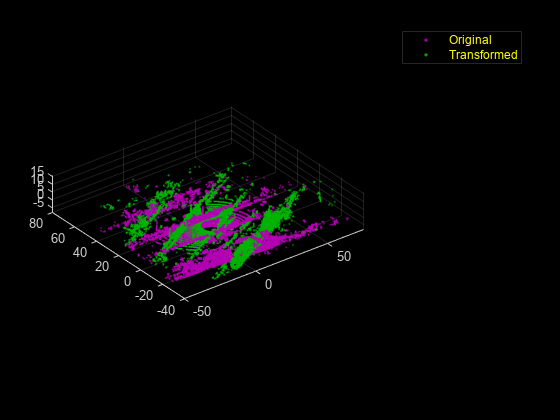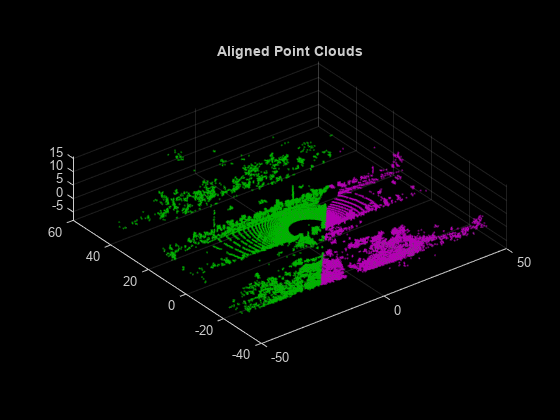# Estimate Transformation Between Two Point Clouds Using Features

This example shows how to estimate rigid transformation between two point clouds. In the example, you use feature extraction and matching to significantly reduce the number of points required for estimation. After you use the `extractFPFHFeatures` function to extract fast point feature histogram (FPFH) features from the point clouds, you use the `pcmatchfeatures` function to search for matches in the extracted features. Finally, you use tge `estimateGeometricTransform3D` function and the matching features to estimate the rigid transformation.

### Preprocessing

Create two point clouds by applying rigid transformation to an input point cloud.

Read the point cloud data into the workspace.

```rng("default") ptCld = pcread("highwayScene.pcd"); ptCld.Count```
```ans = 65536 ```

Downsample the point cloud to improve the computation speed, as it contains around 65,000 points.

```ptCloud = pcdownsample(ptCld,"gridAverage",0.2); ptCloud.Count```
```ans = 24596 ```

Create a rigid transformation matrix with a 30-degree rotation and translation of 5 units in x- and y-axes.

```rotAngle = 30; rot = [cosd(rotAngle) sind(rotAngle) 0; ... -sind(rotAngle) cosd(rotAngle) 0; ... 0 0 1]; trans = [5 5 0]; tform = rigid3d(rot,trans);```

Transform the input point cloud.

`ptCloudTformed = pctransform(ptCloud,tform);`

Visualize the two point clouds.

```pcshowpair(ptCloud,ptCloudTformed) xlim([-50 75]) ylim([-40 80]) legend("Original", "Transformed","TextColor",[1 1 0])```### Feature Extraction and Registration

Extract features from both the point clouds using the `extractFPFHFeatures` function.

```fixedFeature = extractFPFHFeatures(ptCloud); movingFeature = extractFPFHFeatures(ptCloudTformed);```

Find matching features and display the number of matching pairs.

```[matchingPairs,scores] = pcmatchfeatures(fixedFeature,movingFeature, ... ptCloud,ptCloudTformed,"Method","Exhaustive"); length(matchingPairs)```
```ans = 1814 ```

Select matching points from the point clouds.

```fixedPts = select(ptCloud,matchingPairs(:,1)); matchingPts = select(ptCloudTformed,matchingPairs(:,2));```

Estimate the transformation matrix using the matching points.

```estimatedTform = estimateGeometricTransform3D(fixedPts.Location, ... matchingPts.Location,"rigid"); disp(estimatedTform.T)```
``` 0.8660 0.5000 0.0002 0 -0.5000 0.8660 -0.0002 0 -0.0003 0.0000 1.0000 0 4.9995 5.0022 0.0020 1.0000 ```

Display the defined transformation matrix.

`disp(tform.T)`
``` 0.8660 0.5000 0 0 -0.5000 0.8660 0 0 0 0 1.0000 0 5.0000 5.0000 0 1.0000 ```

Use the estimated transformation to retransform `ptCloudTformed` back to the initial point cloud.

`ptCloudTformed = pctransform(ptCloudTformed,invert(estimatedTform));`

Visualize the two point clouds.

```pcshowpair(ptCloud,ptCloudTformed) xlim([-50 50]) ylim([-40 60]) title("Aligned Point Clouds")```## SupportGet trial now